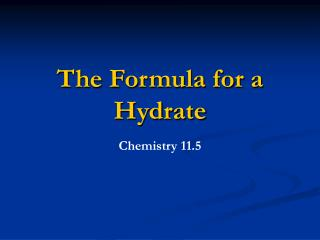DownloadDownload PresentationThe Formula for a Hydrate

# The Formula for a Hydrate

Télécharger la présentation## The Formula for a Hydrate

- - - - - - - - - - - - - - - - - - - - - - - - - - - E N D - - - - - - - - - - - - - - - - - - - - - - - - - - -
##### Presentation Transcript

1. The Formula for a Hydrate Chemistry 11.5

2. Objectives • Explain what a hydrate is and how its name reflects is composition. • Determine the formula for a hydrate.

3. Key Terms • Hydrate

4. Naming Hydrates • A compound that has a specific number of water molecules bound to its atoms. • The number of water molecules is written following a dot. • Na2CO3 • 10 H2O • sodium carbonate decahydrate (Table 11-1) • Note: When calculating molar mass, the mass of water associated with the formula must be included.

5. Analyzing a Hydrate • To analyze a hydrate, the water associated with the compound must be driven off. • Usually this is done by heating the material. • The remaining substance is termed anhydrous: “without water.”

6. Uses of Hydrates • Absorb water when in the anhydrous form. • Added to fuel to keep water out the of mixture. • Keeps electronics safe from humid air conditions.

7. Formula for a Hydrate • Determine the number of moles of water associated with one mole of the hydrate. • Example: Suppose you have a 5.00 g sample of a hydrate of barium chloride. • Formula is BaCl2 • xH2O. • After heating substance until water is driven off, mass is 4.26 g.

8. Formula for a Hydrate • 5.00 g BaCl2 hydrate – 4.26 g anhydrous BaCl2 = 0.74 g H2O. • Now convert the grams to mol. • 4.26 g BaCl2 x 1 mol BaCl2 = 0.0205 mol BaCl2 208.23 g BaCl2 • 0.74 g H2O x 1 mol H2O = 0.041 mol H2O 18.02 g H2O

9. Formula for a Hydrate • To determine the coefficient that precedes water in the formula for a hydrate: • (moles water/moles compound) • 0.041 mol H2O = 2 0.0205 mol BaCl2 • BaCl2 • 2 H2O

10. Practice Problems • Pg 340

11. Homework • Section 11.5 Problems 33 and 34 on page 877